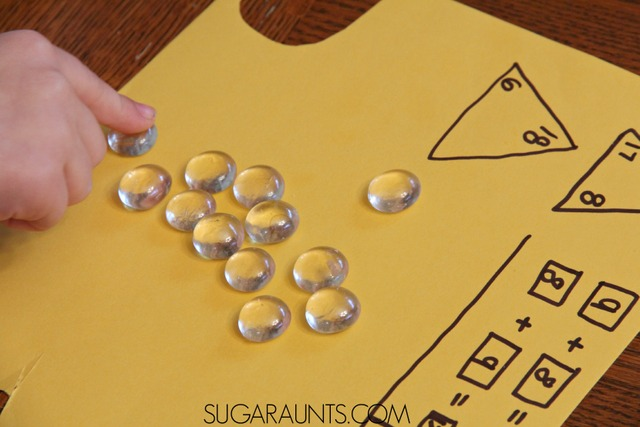How to Compose and Decompose Numbers | The OT Toolbox

## How to Compose and Decompose Numbers

Most of us have glass gems sitting around the house.  (Don't have any?  See where we got them super cheap below!)  These glass gems came in handy when we were practicing first grade math recently.  Math and common core standards can be a tricky thing with terms like composing and decomposing numbers.  They can throw us old moms and dads for a loop when we are helping out with homework duty (or following guidelines in homeschooling curricula).  Today's post for our 31 Days of Learning with Free (or almost free) Materials was a fun and easy way to practice difficult first grade math concepts in a fun way!This post contains affiliate links.

## How do you Compose and Decompose numbers (First Grade Math):

To start with, we used clear glass gemsthat we found in the dollar store.  You can get a pound of gems for a dollar which will go a long way in math and other activities with the kids.  You can quickly purchase the gems here for convenience.## What is "composing numbers"?

To practice our composing and decomposing of numbers, we pulled out a handful of the glass gems and paper with a marker.  I drew a triangle and wrote two numbers in two corners of the triangles.  My oldest daugher (in first grade at the time) used the gems as counting manipulatives.  She added the two numbers up by counting the glass gems and wrote the total in the empty corner of the triangle.   This adding numbers together, or building a number is called composing a number.  Another way to say composing numbers is to think about putting numbers together.  My daughter came home from school talking about how to "put together" numbers.

## What is "decomposing numbers"?

To decompose the number, we wrote another triangle and started with a higher number and a lower number.  She used the clear glass gems to subtract by counting out the manipulatives.  This taking apart of a number is called decomposing a number.  You can think about decomposing numbers by "taking apart" a number.We then wrote out the addition and subtraction sentences using boxes with the composed and decomposed numbers.  It is interesting to see the light bulb go off as your child realizes that a number can be built in many ways, but if you take away a specific amount of gems (or subtract), there is only one number that can "fit" in that corner of the triangle.  Likewise, if you subtract either number from a composed triangle statement, you will always get the other corner's number (assuming the math has been done correctly).

You can extend this activity out more by filling in just one corner of the triangle.  Draw  a page full of triangles.  Ask your child to put together, or compose, that number in as many different ways as they can.
Work on decomposing a number by filling in just one corner of a triangle.  Draw a page full of triangles.  Ask your child to take apart, or decompose, that number in as many different ways as they can.

See all of our Learning with Free (or almost free) Materials.

This post is part of the 31 Days of Homeschool Tips series.

See all of our math posts here.

Love using regular every day items in learning and play?  Get our published book for 150+ activities for kids that can be done throughout the year: